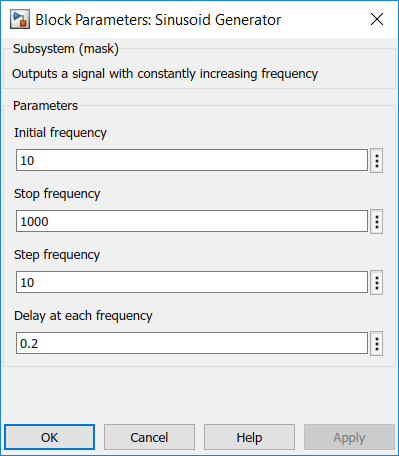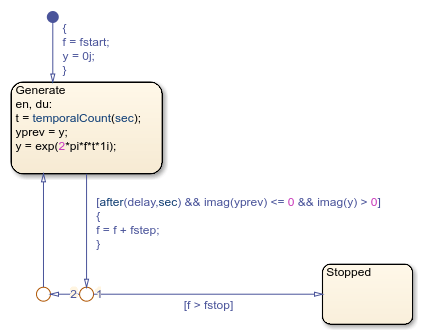Documentation

### This is machine translation

Mouseover text to see original. Click the button below to return to the English version of the page.

## Measuring the Frequency Response of a System

This model shows a method for measuring the frequency response of a continuous time system (plant) using Stateflow®. It illustrates several features of Stateflow such as the `temporalCount` function and change detection. Note that this chart uses MATLAB® as the action language.### Overview

Briefly, the model consists of several stages:

1. A sinusoidal signal generator that produces complex sinusoidal signals of increasing frequency.

2. A second order continuous time system whose frequency response we want to measure.

3. A Stateflow chart (`Analyzer`) that measures the magnitude and phase shift of the response of the continuous system.

4. A Stateflow chart (`Unwrap`) that works like the MATLAB function `unwrap` and wraps the frequency response so that there are no sharp jumps fromto.

### Sinusoid Generator

The sinusoid generator is a subsystem which outputs a complex signal of increasing frequency. For every frequency, the signal persists at that frequency for a given hold time. The subsystem has two outputs:

1. A scalar `f` which is the current frequency

2. A complex signal `y` which is a complex signal with the frequency `f`.

The behavior of the subsystem is parameterized by its mask dialog parameters. For example, for the given values below:the subsystem outputs a sinusoidal signal which starts at 10Hz, holds that frequency for 0.2 seconds and then steps up the frequency by 10Hz. This continues till the frequency reaches 1000Hz.

The sinusoid generator is implemented using a Stateflow chart as below:

Since the chart uses MATLAB as the action language, the logic to compute the complex signal with the current frequency `f` can be directly entered as a state action:

```yprev = y; y = exp(2*pi*f*t*1j); ```

The input to this function is the elapsed time (in seconds) since we last changed the frequency. Note the use of the Stateflow built-in function `temporalCount` which counts the number of seconds elapsed since the state was last entered.

The state `A` is re-entered every time `delay` seconds have elapsed and the imaginary part of the output just crosses zero. This ensures that the signal changes frequency when it has a small imaginary part. Without the extra check, the input to the plant would switch from a high value to zero in two successive time-steps. This would result in the output from the plant taking much longer to stabilize at the new frequency.

### Plant

The plant in this example is a simple second order resonant system. In a real world application, this would be implemented via xPC D/A and A/D blocks which would measure the response of a DUT (Device Under Test).

### Analyzer

The analyzer chart takes as inputs the complex signal which is the input to the plant and the measured (real) output from the plant. It outputs the magnitude and phase of the plant output.

The analyzer chart works by maintaining two running sums for every frequency. The sum `y` is the accumulation (integral) of the product of the plant output (a real number) with the complex signal `u`. The sum `yn` is the accumulation of the product of the plant input with the complex signal `u`. Thus `yn` represents the accumulation of a hypothetical plant with a unit transfer function.

When the frequency switches (which the Analyzer chart detects using the Stateflow built-in function `hasChanged`), it computes the magnitude and phase of the plant output by normalizing `y` with respect to `yn`.

### Unwrap

The `Unwrap` chart mimics the built-in MATLAB function unwrap. Note the use of the combined entry-during action specified using the syntax `en,du:`. Thus the action happens both when the state is first entered as well as for the duration of the activity of the state.

### Simulation Results

When the model is simulated, the magnitude and the phase of the continuous time system can be measured as shown below. We see that the plant achieves resonance around t = 10 seconds.If we plot the measured magnitude and phase against the plant input frequency, we get the measured frequency response or the bode plot for the plant. As can be seen, the measured resonant frequency is around 150Hz with a peak amplitude of around 1.6. This closely matches the plant dynamics which is given by:

``

with

``
``## Related Topics

##### SupportGet trial now